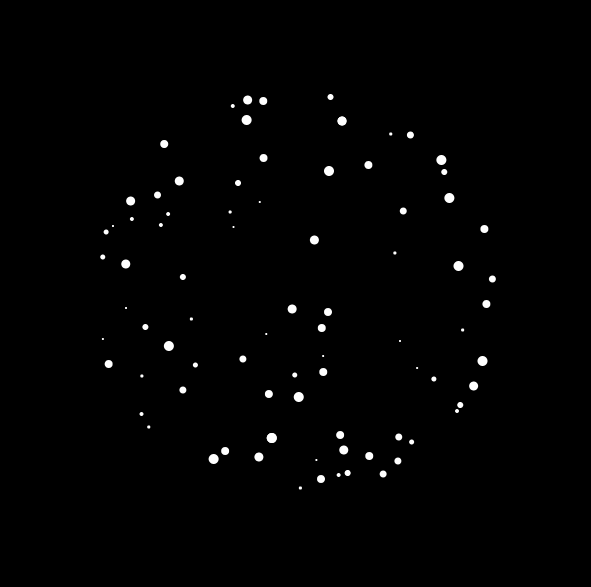#### Howdy, Stranger!

We are about to switch to a new forum software. Until then we have removed the registration on this forum.

# Can you interpret this particle code for me?

edited April 2018

Hello Processing Community,I have used this particle case, but there are a few sentences about abs(), sqrt() can't read, can you help me understand this?

``````    float circleSize = 400;
int period = 5; //speed
float x = 0;
float particleY;
float amplitude;
float sineEl;

int randomPart[] = new int;
int partSize[] = new int;

void setup() {
size(600, 600);
for (int i = 0; i < randomPart.length; i++) {
randomPart[i] = int(random(100, 500));
partSize[i] = int(random(2, 11));
}
}

void draw() {
background(0);
x += 0.01;

for (int yRand = 0; yRand < randomPart.length; yRand++) {

particleY = randomPart[yRand];

float sine = sin((3*PI*x)/period + randomPart[yRand]);//this
amplitude = sqrt(sq(radius) - sq(abs(height/2 - particleY))); // and this
sineEl = width/2 + sine * amplitude;//and this i don't konw

float particleSize = partSize[yRand];
//rotation
pushMatrix();
translate(width/2, height/2);
translate(-width/2, -height/2);
stroke(255);
noStroke();
fill(255);
ellipse(sineEl, particleY, particleSize, particleSize);
println(sineEl, particleY, particleSize, particleSize);
popMatrix();
}

noFill();
stroke(0);
}
``````Tagged:

• edited April 2018 Answer ✓

The sin line is wrapping the points around the sphere, the contents inside the sin() function kind of like a longitude line. This becomes clear if you play with the contents, like changing the line to `float sine = sin((6 * PI * x)/period + 1);` The animation part comes from the x variable which is increased every frame.

The abs() isn't really needed, if you remove it, the effect is still the same. If you remove the sq() wrapping it, the sphere turns into a cylinder.

The third line is multiplying the amplitude(kinda like the lattitude) by the sine(kinda like the longitude) to make it spherical.

• abs etc are explained in the reference